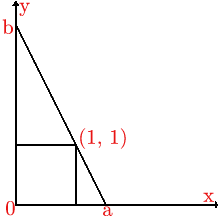#### You may also like### Just Opposite

A and C are the opposite vertices of a square ABCD, and have coordinates (a,b) and (c,d), respectively. What are the coordinates of the vertices B and D? What is the area of the square?### Hypotenuse Lattice Points

The triangle OMN has vertices on the axes with whole number co-ordinates. How many points with whole number coordinates are there on the hypotenuse MN?### Beelines

Is there a relationship between the coordinates of the endpoints of a line and the number of grid squares it crosses?

##### Age 14 to 16Challenge Level

This solution is by Anthony of Prince Henry's High School, Evesham. Well done Anthony.

I viewed the ladder as a line, cutting the $x$ axis at $x = a$ and the $y$ axis at $y = b$, passing through the point $(1,1)$ with $a$ and $b$ such that $a^2 + b^2 = 16$ by Pythagoras theorem. The gradient of the line is $- b/a$ (the change in $y$ divided by the change in $x$). As $a =\sqrt{16-b^2}$, the gradient is $\frac{-b}{\sqrt{16 - b^2}}.$ The equation of the line of the ladder is:

 $$y = -\frac{b}{\sqrt{16 - b^2}}x + b.$$ When $x = 1, \ y = 1$ we have $$1 = \frac{-b}{\sqrt{16 - b^2}} + b$$ and hence $$b^4 - 2b^3 - 14b^2 + 32 b -16 = 0.$$This can be solved using a numerical search method (such as interval halving) to give the approximate solutions $b = 1.36$ and $3.76$ (to $2$ decimal places). These are the two possible heights of the top of the ladder. In each case, if one value is the height of the top of the ladder, the other value is the distance of the foot of the ladder from the wall.

Alternatively, the gradient is $-b/a = (1 - b)/(1 - 0)$, hence $ab = a + b$. Using the fact that $a^2 + b^2 = 16$ this gives $$(ab)^2 = (a + b)^2 = a^2 + 2ab + b^2 = 16 + 2ab.$$ Hence $$(ab)^2 - 2ab - 16 = 0.$$ Solving this equation gives $ab = 1 \pm \sqrt{17}$. As $a$ and $b$ are positive the solution here is $ab = 1 + \sqrt {17}$. We are looking for $a$ and $b$ and we know the sum $a + b$ and the product $ab$ are both equal to $1 + \sqrt{17}$. We can write down a quadratic equation with these roots and solve it. Hence $a$ and $b$ are the roots of $$x^2 - (1 + \sqrt{17})x + (1 + \sqrt{17}) = 0.$$

Solving this equation using the quadratic formula gives the values $3.7609$ and $1.3622$ (to $4$ decimal places). There are two solutions, one with the ladder at a steeper angle (it turns out to be approximately $70$ degrees) and the other at approximately $20$ degrees.

Yet another method uses numerical approximation. Taking the angle of the ladder with the ground to be $\theta$, then $a = 4 \cos \theta$ and $b = 4 \sin \theta$. Set up a spreadsheet or a program to evaluate $ab - (a + b)$ and use an interval halving method to find value of $\theta$ for which $ab - (a + b)$ is close enough to zero to give the values of $a$ and $b$ to sufficient accuracy.